## Help Centre

ASHAVI SURVEY contains 20 question types include standard question types such as single choice, multiple choice, etc, and more advance data collection types such as image data, video clip data, etc. ASHAVI SURVEY enable you to conduct the good survey questionnaire and gain insight easily1. Single choice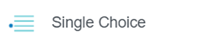This is the type of sentence where the respondent can only choose 1 answer

The sample question is as follows: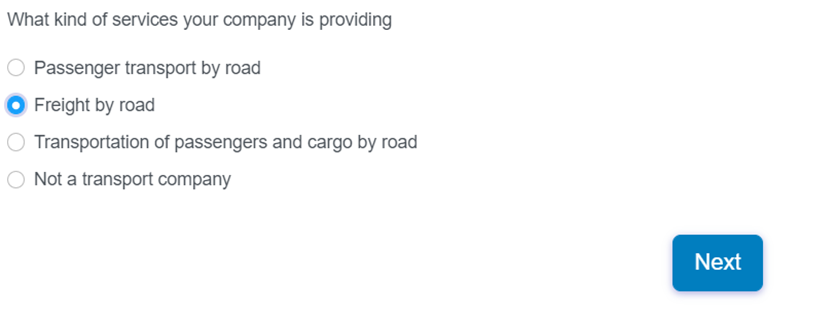2. Single Choice Grid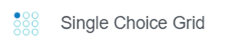This is a form with many sentences where respondents can only choose 1 answer per sentence.

The sample question is as follows: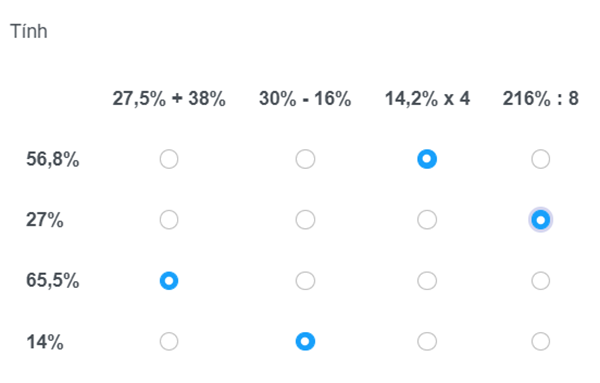3. Multiple ChoiceThis is the type of sentence where the respondent is selected for many answers.

The sample question is as follows: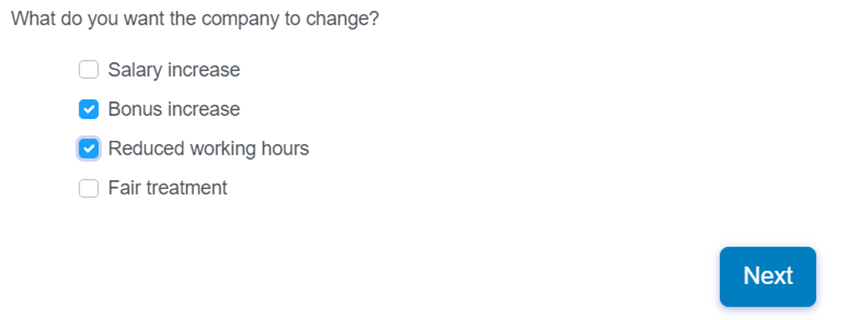4. Multiple Chice Grid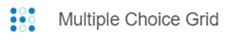This is a form with many sentences where respondents are given many answers.

The sample question is as follows: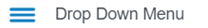This is the type of sentence where the respondent is given 1 unique answer.

The sample question is as follows:This is a form with 1 question of the same type where the answer is given a unique answer for each small idea.

The sample question is as follows: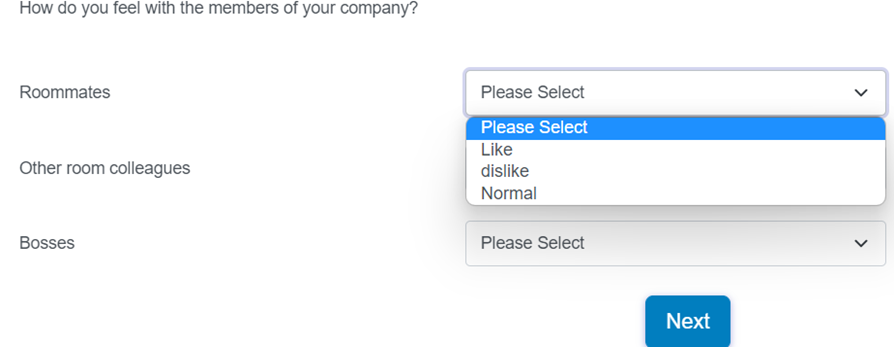This is a form with many questions of the same type that the respondent chooses 1 unique answer for each sentence

The sample question is as follows: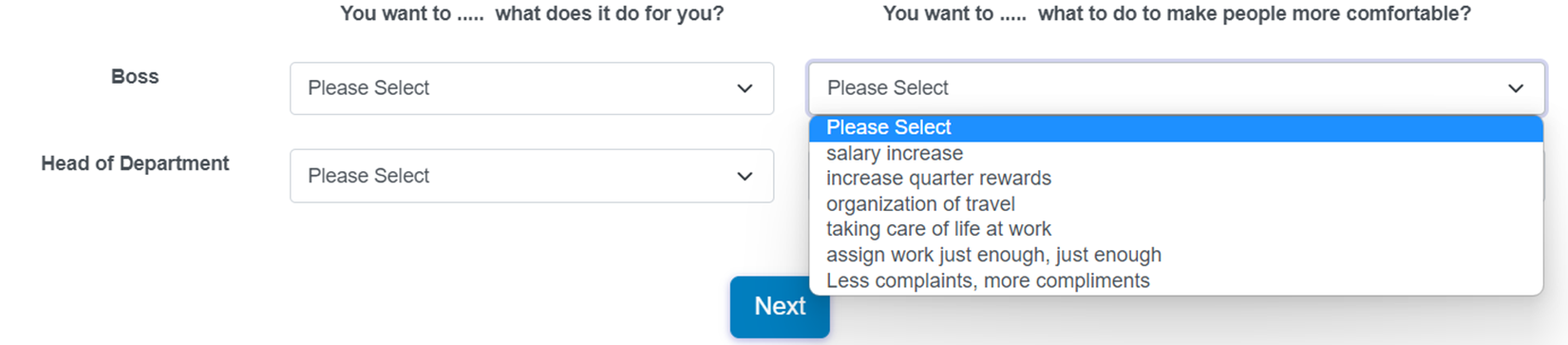8. Text boxThis is the type of sentence that the respondent will fill in the answer in the box below.

The sample question is as follows: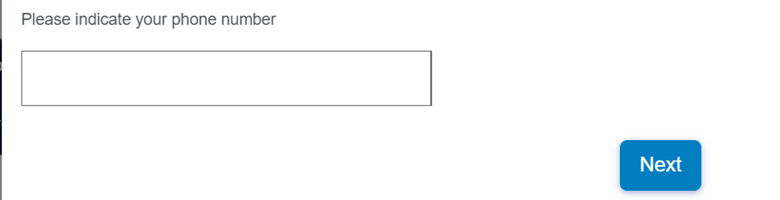9. Long text boxThis is the type of sentence that the respondent will manually fill in the answer in the box below - for writing long paragraphs.

The sample question is as follows:10. Date/ Month/ Year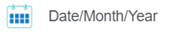This is the type of sentence where respondents will choose the appropriate date according to the available calendar.

The sample question is as follows: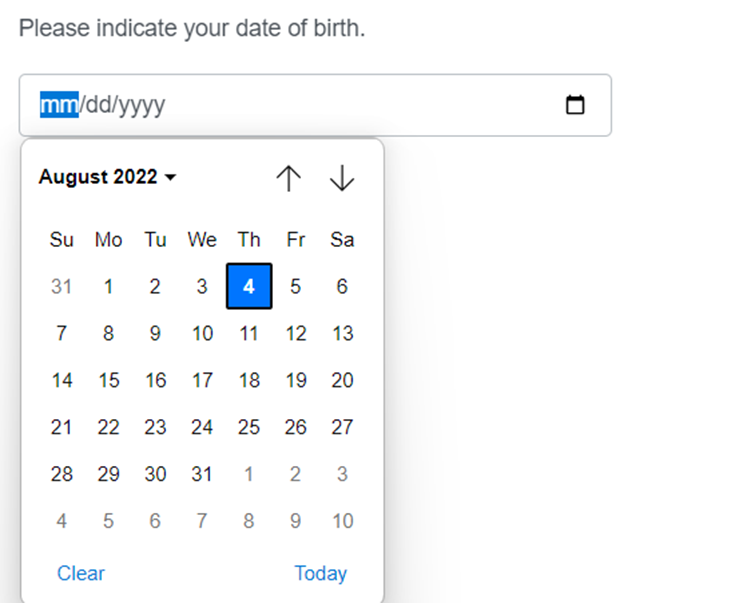11. Rating Star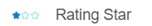This is the type of sentence in which the respondent will choose the degree corresponding to the given number of stars

The sample question is as follows: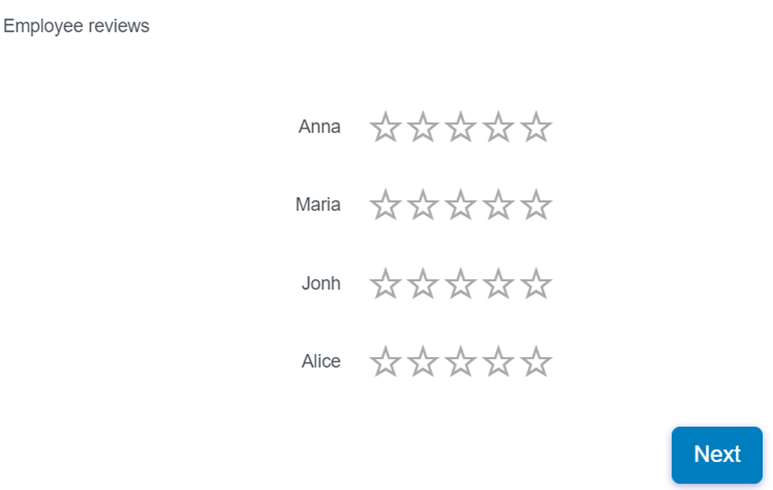12. Rating Star GridThis is a form of sentence with many overlapping ideas that respondents only need to choose the appropriate level for each idea

The sample question is as follows:13. Silder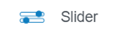This is the type of sentence that respondents only need to answer by dragging the slide on the long bar.

The sample question is as follows: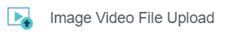This is the type of sentence where respondents will upload images or videos as required.

The sample question is as follows: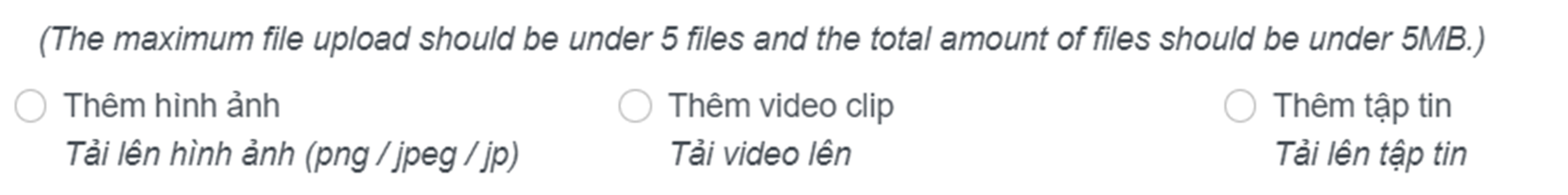15. Image Single ChoiceThis is the type of sentence where respondents can select multiple images

The sample question is as follows: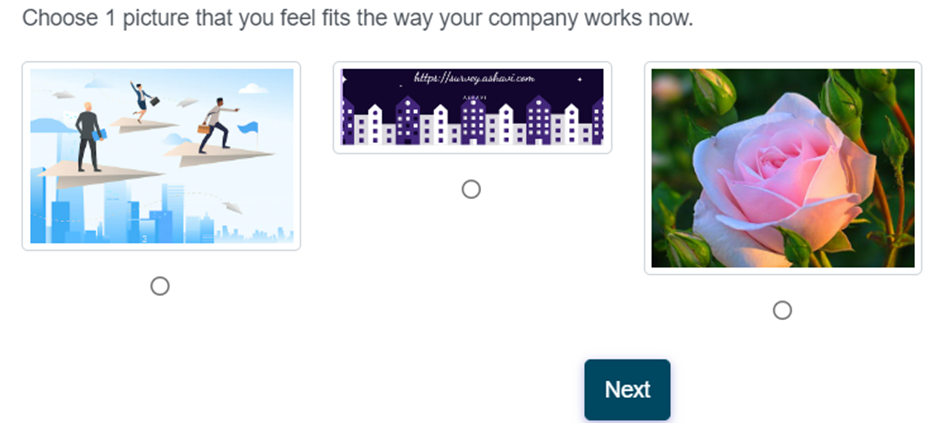16.Image Multiple ChoiceThis is the type of sentence where respondents can select multiple images

The sample question is as follows:17. Drag and Drop RankingThis is the sentence where the respondent will drag the answer from the left box to the right box.

The sample question is as follows:18. Continuous Sum TotalThis is the type of sentence where the respondent will find the sum of the values

The sample question is as follows:19. Grouping CardsThis is the type of sentence where the respondent will drag the selection boxes into the corresponding columns.

The sample question is as follows:20. RX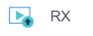This is the type of sentence where respondents will add images or videos as required.

The sample question is as follows:Help Centre: